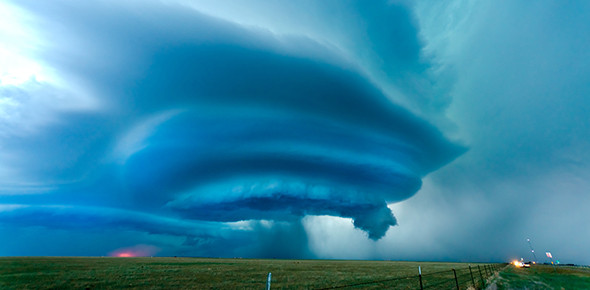# Physical Meteorology Test 1

29 Questions | Attempts: 383
ShareSettingsQuestions on beginner topics in Physical Meteorology.

• 1.
Earth's dry atmosphere is mainly made up of which gasses?
• A.

Oxygen

• B.

Nitrogen

• C.

Carbon Dioxide

• D.

Argon

• E.

Methane

• 2.
What percentage is Earth's atmosphere made up of Oxygen?
• A.

80%

• B.

78%

• C.

23%

• D.

21%

• 3.
In accordance with the Gas Law, if V is doubled, then P is
• A.

Halved

• B.

Doubled

• C.

Not affected

• 4.
Earth's atmosphere is made up of what percentage of Nitrogen?
• A.

81%

• B.

78%

• C.

21%

• D.

1%

• 5.
According to the Gas Law, if T is doubled, then V is
• A.

Halved

• B.

Doubled

• C.

Not affected

• 6.
In accordance with the Gas Law, if T is doubled, then P is
• A.

Halved

• B.

Doubled

• C.

Not affected

• 7.
The value of R* is _______.
• 8.
The Gas constant, R, for any atmosphere can be found by dividing R* by the sum of the products of the ___________ .
• 9.
The Hypsometric equation relates height to temperature and can be written out as follows: ______ .∫ integral symbol if needed
• 10.
Dq = dw + dudq = pdv + dudq = pdα + dudq = pdα + CVdTdq = CPdT - αdpdq = dh - αdpdq = d(CPT + ϕ)These are forms of what Law?
• 11.
Complete the Ideal Gas law:__ = ρRT
• 12.
Complete the Ideal Gas Law:pv = n__T
• 13.
Complete the Ideal Gas Law:p__ = mRT
• 14.
Work is "path dependent."
• A.

True

• B.

False

• 15.
The equation for Work is ∫ integral symbol if needed
• 16.
What are common paths for work? Check all that apply.
• A.

Isobaric

• B.

Isovolumetric

• C.

Isothermal

• D.

• 17.
When the parcel expands, work is being done on the parcel.
• A.

True

• B.

False

• 18.
What is enthalpy a measure of?
• 19.
What is CPT + ϕ ?
• A.

Geopotential Height

• B.

Dry Static Energy

• C.

Constant Pressure

• D.

Specific Heat of Pressure

• 20.
Which describe a parcel?A parcel is some piece of air with an indeterminate volume and mass that:
• A.

Is thermally insulated from its environment

• B.

Assumes the same pressure as its environment

• C.

Moves slow enough that its kinetic energy is small compared to its internal energy

• D.

All of the above

• 21.
To get the Dry Adiabatic Lapse Rate, you go from:0 = dq = d(CPT + ϕ) to (dT/dz) = (-g/CP) = -9.8 k/km
• A.

True

• B.

False

• 22.
• A.

No pressure exchange with environment

• B.

No heat exchange with environment

• C.

Conserves Dry Static Energy

• D.

Conserves Potential Temperature

• 23.
What is potential temperature?
• A.

The temperature a parcel could have when it puts its mind to it.

• B.

The temperature a parcel could have when it is moved , dry adiabatically, to 850 mb.

• C.

The temperature stored inside of a parcel.

• D.

The temperature a parcel could have when it is moved, dry adiabatically, to 1000 mb.

• 24.
This equation:θ = T(1000mb/p)^(R/CP)Is for what value?
• A.

Potential Temperature

• B.

Lapse Rate

• C.

Geopotential Height

• D.

Specific Volume

• E.

Density

• 25.
What are good criteria for a useful thermodynamic diagram?
• A.

Maximum number of straight lines

• B.

Coordinates to be measured

• C.

Vertical coordinate to be approximately height

• D.

Adiabats and isotherms to be parallel

• E.

Area to be proportional to energy

## Related TopicsBack to top
×

Wait!
Here's an interesting quiz for you.